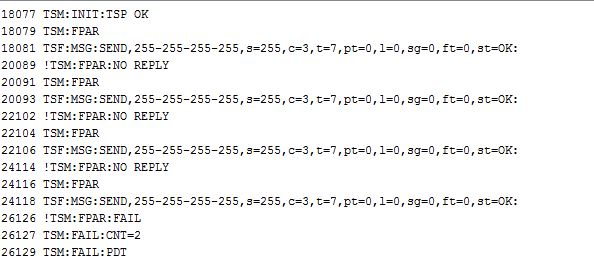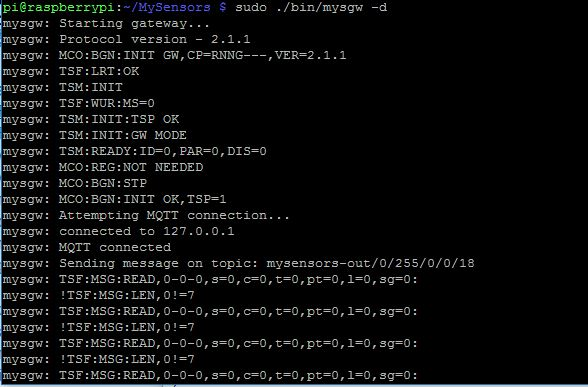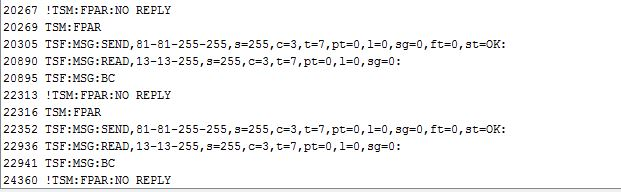# Rpi3 GW, Nano, NRF24L01+ not connecting.

• Hi everyone,
I am setting up my first sensor network. I have a Raspi 3 running OH2 and MQTT gateway. That part went well. I can subscribe to mqtt messages, and see the gateway come on line and connect. So far so good. I am trying to create a simple relay actuator sensor. I wired some NRF24L01+ radios to a couple of Arduino Nanos. But with each nano I see the followingand the gateway showsI measured the power, 3.3v to the radios. I've tried changing power sources, but it's the same. The gateway and the sensors are sitting on the same bench a foot away from each other.

Can anyone suggest what to try next?

• I think you missed to set up node ID on the 2 Nano, but I don't see the complete log.

• @leitefrog quick addition, if I add two sensors (#13 and #81), they appear to see each other. The serial monitor shows• Pls add the log as text with the code TAG, don't post screenshot. Also post the log from the beginning and if possible the code you are using

• Thanks for the heads up.
I rebooted everything to get a clean log. It acted a bit different.
Here is the sensor log.

``````0 MCO:BGN:INIT REPEATER,CP=RNNRA--,VER=2.1.1
3 MCO:BGN:BFR
b4 5 TSM:INIT
6 TSF:WUR:MS=0
13 TSM:INIT:TSP OK
14 TSM:INIT:STATID=13
16 TSF:SID:OK,ID=13
18 TSM:FPAR
55 TSF:MSG:SEND,13-13-255-255,s=255,c=3,t=7,pt=0,l=0,sg=0,ft=0,st=OK:
440 TSF:MSG:BC
2064 TSM:FPAR
2100 TSF:MSG:SEND,13-13-255-255,s=255,c=3,t=7,pt=0,l=0,sg=0,ft=0,st=OK:
2488 TSF:MSG:BC
4110 TSM:FPAR
4146 TSF:MSG:SEND,13-13-255-255,s=255,c=3,t=7,pt=0,l=0,sg=0,ft=0,st=OK:
4538 TSF:MSG:BC
6156 TSM:FPAR
6192 TSF:MSG:SEND,13-13-255-255,s=255,c=3,t=7,pt=0,l=0,sg=0,ft=0,st=OK:
8200 !TSM:FPAR:FAIL
8201 TSM:FAIL:CNT=1
8203 TSM:FAIL:PDT
18206 TSM:FAIL:RE-INIT
18208 TSM:INIT
18215 TSM:INIT:TSP OK
18217 TSM:INIT:STATID=13
18220 TSF:SID:OK,ID=13
18222 TSM:FPAR
18258 TSF:MSG:SEND,13-13-255-255,s=255,c=3,t=7,pt=0,l=0,sg=0,ft=0,st=OK:
18652 TSF:MSG:BC
20269 TSM:FPAR
20305 TSF:MSG:SEND,13-13-255-255,s=255,c=3,t=7,pt=0,l=0,sg=0,ft=0,st=OK:
20699 TSF:MSG:BC
22316 TSM:FPAR
22352 TSF:MSG:SEND,13-13-255-255,s=255,c=3,t=7,pt=0,l=0,sg=0,ft=0,st=OK:
22746 TSF:MSG:BC
24363 TSM:FPAR
24399 TSF:MSG:SEND,13-13-255-255,s=255,c=3,t=7,pt=0,l=0,sg=0,ft=0,st=OK:
26407 !TSM:FPAR:FAIL
26408 TSM:FAIL:CNT=2
26411 TSM:FAIL:PDT
36414 TSM:FAIL:RE-INIT
36416 TSM:INIT
36423 TSM:INIT:TSP OK
36425 TSM:INIT:STATID=13
36427 TSF:SID:OK,ID=13
36429 TSM:FPAR
36466 TSF:MSG:SEND,13-13-255-255,s=255,c=3,t=7,pt=0,l=0,sg=0,ft=0,st=OK:
36583 TSF:MSG:FPAR OK,ID=0,D=1
36859 TSF:MSG:BC
38473 TSM:FPAR:OK
38474 TSM:ID
38476 TSM:ID:OK
38477 TSM:UPL
38514 !TSF:MSG:SEND,13-13-0-0,s=255,c=3,t=24,pt=1,l=1,sg=0,ft=0,st=NACK:1
38906 TSF:MSG:BC
40522 TSM:UPL
40559 !TSF:MSG:SEND,13-13-0-0,s=255,c=3,t=24,pt=1,l=1,sg=0,ft=1,st=NACK:1
40953 TSF:MSG:BC
42566 TSM:UPL
42603 !TSF:MSG:SEND,13-13-0-0,s=255,c=3,t=24,pt=1,l=1,sg=0,ft=2,st=NACK:1
44610 TSM:UPL
44647 !TSF:MSG:SEND,13-13-0-0,s=255,c=3,t=24,pt=1,l=1,sg=0,ft=3,st=NACK:1
46654 !TSM:UPL:FAIL
46655 TSM:FPAR
46692 TSF:MSG:SEND,13-13-255-255,s=255,c=3,t=7,pt=0,l=0,sg=0,ft=4,st=OK:
48702 TSM:FPAR
48739 TSF:MSG:SEND,13-13-255-255,s=255,c=3,t=7,pt=0,l=0,sg=0,ft=0,st=OK:
50748 TSM:FPAR
50785 TSF:MSG:SEND,13-13-255-255,s=255,c=3,t=7,pt=0,l=0,sg=0,ft=0,st=OK:
52794 TSM:FPAR
52831 TSF:MSG:SEND,13-13-255-255,s=255,c=3,t=7,pt=0,l=0,sg=0,ft=0,st=OK:
53021 TSF:MSG:BC
54838 !TSM:FPAR:FAIL
54839 TSM:FAIL:CNT=3
54841 TSM:FAIL:PDT
64844 TSM:FAIL:RE-INIT
64846 TSM:INIT
64854 TSM:INIT:TSP OK
64856 TSM:INIT:STATID=13
64858 TSF:SID:OK,ID=13
64860 TSM:FPAR
64897 TSF:MSG:SEND,13-13-255-255,s=255,c=3,t=7,pt=0,l=0,sg=0,ft=0,st=OK:
66906 TSM:FPAR
66942 TSF:MSG:SEND,13-13-255-255,s=255,c=3,t=7,pt=0,l=0,sg=0,ft=0,st=OK:
68952 TSM:FPAR
68988 TSF:MSG:SEND,13-13-255-255,s=255,c=3,t=7,pt=0,l=0,sg=0,ft=0,st=OK:
70999 TSM:FPAR
71035 TSF:MSG:SEND,13-13-255-255,s=255,c=3,t=7,pt=0,l=0,sg=0,ft=0,st=OK:
73043 !TSM:FPAR:FAIL
73044 TSM:FAIL:CNT=4
73047 TSM:FAIL:PDT
83050 TSM:FAIL:RE-INIT
83052 TSM:INIT
83059 TSM:INIT:TSP OK
83061 TSM:INIT:STATID=13
83063 TSF:SID:OK,ID=13
83065 TSM:FPAR
83102 TSF:MSG:SEND,13-13-255-255,s=255,c=3,t=7,pt=0,l=0,sg=0,ft=0,st=OK:
85111 TSM:FPAR
85148 TSF:MSG:SEND,13-13-255-255,s=255,c=3,t=7,pt=0,l=0,sg=0,ft=0,st=OK:
85762 TSF:MSG:FPAR OK,ID=0,D=1
87155 TSM:FPAR:OK
87156 TSM:ID
87158 TSM:ID:OK
87159 TSM:UPL
87196 !TSF:MSG:SEND,13-13-0-0,s=255,c=3,t=24,pt=1,l=1,sg=0,ft=0,st=NACK:1
87208 TSF:MSG:PONG RECV,HP=1
87212 TSM:UPL:OK
87252 !TSF:MSG:SEND,13-13-0-0,s=255,c=3,t=15,pt=6,l=2,sg=0,ft=0,st=NACK:0100
89296 !TSF:MSG:SEND,13-13-0-0,s=255,c=0,t=18,pt=0,l=5,sg=0,ft=1,st=NACK:2.1.1
89339 !TSF:MSG:SEND,13-13-0-0,s=255,c=3,t=6,pt=1,l=1,sg=0,ft=2,st=NACK:0
91384 !TSF:MSG:SEND,13-13-0-0,s=255,c=3,t=11,pt=0,l=5,sg=0,ft=3,st=NACK:Relay
91428 !TSF:MSG:SEND,13-13-0-0,s=255,c=3,t=12,pt=0,l=3,sg=0,ft=4,st=NACK:1.0
91470 !TSF:MSG:SEND,13-13-0-0,s=1,c=0,t=3,pt=0,l=0,sg=0,ft=5,st=NACK:
91512 !TSF:MSG:SEND,13-13-0-0,s=2,c=0,t=3,pt=0,l=0,sg=0,ft=6,st=NACK:
91518 MCO:REG:REQ
91556 !TSF:MSG:SEND,13-13-0-0,s=255,c=3,t=26,pt=1,l=1,sg=0,ft=7,st=NACK:2
93534 TSF:MSG:BC
93535 TSF:MSG:FPAR REQ,ID=81
93538 TSF:CKU:OK,FCTRL
93540 TSF:MSG:GWL OK
93901 TSF:MSG:SEND,13-13-81-81,s=255,c=3,t=8,pt=1,l=1,sg=0,ft=8,st=OK:1
93944 !TSF:MSG:SEND,13-13-0-0,s=255,c=3,t=26,pt=1,l=1,sg=0,ft=8,st=NACK:2
95584 TSF:MSG:REL MSG
95587 TSF:MSG:REL PxNG,HP=1
95625 !TSF:MSG:SEND,81-13-0-0,s=255,c=3,t=24,pt=1,l=1,sg=0,ft=9,st=NACK:2
95985 !TSF:MSG:SEND,13-13-0-0,s=255,c=3,t=26,pt=1,l=1,sg=0,ft=10,st=NACK:2
95995 TSM:FPAR
96032 TSF:MSG:SEND,13-13-255-255,s=255,c=3,t=7,pt=0,l=0,sg=0,ft=11,st=OK:
97992 !TSF:SND:TNR
98041 TSM:FPAR
98078 TSF:MSG:SEND,13-13-255-255,s=255,c=3,t=7,pt=0,l=0,sg=0,ft=0,st=OK:
101996 TSM:FPAR
102033 TSF:MSG:SEND,13-13-255-255,s=255,c=3,t=7,pt=0,l=0,sg=0,ft=0,st=OK:
103655 TSF:MSG:BC
104042 TSM:FPAR
104079 TSF:MSG:SEND,13-13-255-255,s=255,c=3,t=7,pt=0,l=0,sg=0,ft=0,st=OK:
105699 TSF:MSG:BC
106086 !TSM:FPAR:FAIL
106088 TSM:FAIL:CNT=1
106090 TSM:FAIL:PDT
116093 TSM:FAIL:RE-INIT
116096 TSM:INIT
116103 TSM:INIT:TSP OK
116105 TSM:INIT:STATID=13
116107 TSF:SID:OK,ID=13
116110 TSM:FPAR
116147 TSF:MSG:SEND,13-13-255-255,s=255,c=3,t=7,pt=0,l=0,sg=0,ft=0,st=OK:
118156 TSM:FPAR
118193 TSF:MSG:SEND,13-13-255-255,s=255,c=3,t=7,pt=0,l=0,sg=0,ft=0,st=OK:
120202 TSM:FPAR
120239 TSF:MSG:SEND,13-13-255-255,s=255,c=3,t=7,pt=0,l=0,sg=0,ft=0,st=OK:
121864 TSF:MSG:BC
122248 TSM:FPAR
122285 TSF:MSG:SEND,13-13-255-255,s=255,c=3,t=7,pt=0,l=0,sg=0,ft=0,st=OK:
123912 TSF:MSG:BC
124292 !TSM:FPAR:FAIL
124294 TSM:FAIL:CNT=2
124296 TSM:FAIL:PDT
134299 TSM:FAIL:RE-INIT
134301 TSM:INIT
134308 TSM:INIT:TSP OK
134310 TSM:INIT:STATID=13
134312 TSF:SID:OK,ID=13
134316 TSM:FPAR
134352 TSF:MSG:SEND,13-13-255-255,s=255,c=3,t=7,pt=0,l=0,sg=0,ft=0,st=OK:
136363 TSM:FPAR
136399 TSF:MSG:SEND,13-13-255-255,s=255,c=3,t=7,pt=0,l=0,sg=0,ft=0,st=OK:
138409 TSM:FPAR
138446 TSF:MSG:SEND,13-13-255-255,s=255,c=3,t=7,pt=0,l=0,sg=0,ft=0,st=OK:
140455 TSM:FPAR
140492 TSF:MSG:SEND,13-13-255-255,s=255,c=3,t=7,pt=0,l=0,sg=0,ft=0,st=OK:
142499 !TSM:FPAR:FAIL
142501 TSM:FAIL:CNT=3
142503 TSM:FAIL:PDT
``````

The sketch I took from the examples in the library.

``````/**
* The MySensors Arduino library handles the wireless radio link and protocol
* between your home built sensors/actuators and HA controller of choice.
* The sensors forms a self healing radio network with optional repeaters. Each
* repeater and gateway builds a routing tables in EEPROM which keeps track of the
* network topology allowing messages to be routed to nodes.
*
* Copyright (C) 2013-2015 Sensnology AB
* Full contributor list: https://github.com/mysensors/Arduino/graphs/contributors
*
* Documentation: http://www.mysensors.org
* Support Forum: http://forum.mysensors.org
*
* This program is free software; you can redistribute it and/or
* modify it under the terms of the GNU General Public License
*
*******************************
*
* REVISION HISTORY
* Version 1.0 - Henrik Ekblad
*
* DESCRIPTION
* Example sketch showing how to control physical relays.
* This example will remember relay state after power failure.
* http://www.mysensors.org/build/relay
*/

// Enable debug prints to serial monitor
#define MY_DEBUG

#define MY_NODE_ID 81
// Enable and select radio type attached

// Enable repeater functionality for this node
#define MY_REPEATER_FEATURE

#include <MySensors.h>

#define RELAY_1  3  // Arduino Digital I/O pin number for first relay (second on pin+1 etc)
#define NUMBER_OF_RELAYS 2 // Total number of attached relays
#define RELAY_ON 1  // GPIO value to write to turn on attached relay
#define RELAY_OFF 0 // GPIO value to write to turn off attached relay

void before()
{
for (int sensor=1, pin=RELAY_1; sensor<=NUMBER_OF_RELAYS; sensor++, pin++) {
// Then set relay pins in output mode
pinMode(pin, OUTPUT);

}
}

void setup()
{

}

void presentation()
{
// Send the sketch version information to the gateway and Controller
sendSketchInfo("Relay", "1.0");

for (int sensor=1, pin=RELAY_1; sensor<=NUMBER_OF_RELAYS; sensor++, pin++) {
// Register all sensors to gw (they will be created as child devices)
present(sensor, S_BINARY);
}
}

void loop()
{

}

{
// We only expect one type of message from controller. But we better check anyway.
if (message.type==V_STATUS) {
// Change relay state
digitalWrite(message.sensor-1+RELAY_1, message.getBool()?RELAY_ON:RELAY_OFF);
// Store state in eeprom
saveState(message.sensor, message.getBool());
// Write some debug info
Serial.print("Incoming change for sensor:");
Serial.print(message.sensor);
Serial.print(", New status: ");
Serial.println(message.getBool());
}
}
``````

• Did you enable the repeater for a reason? Do you also have a log of the gateway?

• Thanks again for the help. I went to get the gateway log as you questioned, and noticed that the MQTT was dropping and reconnecting pretty regularly, just as I would see messages coming across. I decided to try using the Ethernet Gateway, so I recompiled, and reinstalled. With that I got things to start working. I have a relay turning on and off from the OpenHab UI. Very cool. Now I'm trying to figure out how to use a two channel relay, but I'll start to search for that.

This is a very helpful forum, and again thanks. I really like the log parser too.
-George

• Regarding the mqtt disconnecting, there was already another user with same problem and, if I remember well, the problem was the client id that was conflicting with another device

3

4

4

5

12

2

3

7